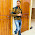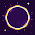# Java code to search an element using Linear Search or Binary Search

/*
Program to search for a number in an array entered by the user using
either Linear Search or Binary Search.
*/
import java.io.*;
class searchArray
{
int a[];
int n;
public searchArray(int nn) // Constructor
{
a = new int[nn];
n = nn;
}
public static void main(String args[]) throws IOException
{
System.out.print("\nEnter the size of the array : ");
int nn = Integer.parseInt(br.readLine());
searchArray call = new searchArray(nn);
System.out.println("\nEnter " +nn +" elements :");
// Ask for the search technique
System.out.println("Choose Search Technique :\n");
System.out.println("1 : Linear Search");
System.out.println("2 : Binary Search (the array should be
sorted in ascending order)");
System.out.print("\nYour Choice : ");
int choice = Integer.parseInt(br.readLine());
int v;
switch(choice)
{
case 1:
System.out.print("\nEnter the number to be searched : ");
call.linearSearch(v);
break;
case 2:
System.out.print("\nEnter the number to be searched : ");
call.binarySearch(v);
break;
default :
System.out.println("\nInvalid Choice !");
break;
}
}
public void readArray() throws IOException
{
for(int i=0;i<n;i++)
}
public void linearSearch(int v)
{
int f=-1;
for(int i=0;i<n;i++)
{
if(a[i]==v)
{
f=i;
break;
}
}
if(f==-1)
else
System.out.println("\n" +v +" is in location " +f);
}
public void binarySearch(int v)
{
int f=-1;
int l=0,m=0,u=n-1;
while(l<=u && f==-1)
{
m = (l+u)/2;
if(a[m]==v)
f = m;
else
if(a[m]>v)
u = m-1;
else
l = m+1;
}
if(f==-1)
else
System.out.println("\n" +v +" is in location " +m);
}
}
/**
* Algorithm for Linear Search :
* ---------------------------
* 1. Start
* 2. Construct a single-dimensional array of appropriate size.
* 3. Fill the array with data entries.
* 4. Ask the user for the number to be searched
* 5. Use linear search technique.
* 6. Print a message whether the number is present in the array or
not.
* 7. End
*
* Algorithm for Binary Search :
* ---------------------------
* 1. Start
* 2. Construct a single-dimensional array of appropriate size.
* 3. Fill the array with data entries in ascending order.
* 4. Ask the user for the number to be searched.
* 5. Use binary search technique.
* 6. Print a message whether the number is present in the array or
not.
* 7. End
*/
/*
Enter the size of the array : 5
Enter 5 elements :
46
324
846
54
541
Choose Search Technique :
1 : Linear Search
2 : Binary Search (the array should be sorted in ascending order)
Your Choice : 1
Enter the number to be searched : 54
54 is in location 3
*/

1.This comment has been removed by the author.

2.This comment has been removed by a blog administrator.

1.Thanks for suggestion...but here you can't promote your site. if you want to promote your site than you can buy small area of this site within small budget..thanks.

3.This comment has been removed by a blog administrator.

4.Nice article about Java linear search program .